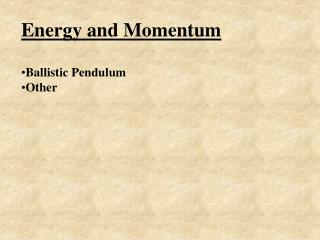DownloadDownload PresentationEnergy and Momentum Ballistic Pendulum Other

# Energy and Momentum Ballistic Pendulum Other

Télécharger la présentation## Energy and Momentum Ballistic Pendulum Other

- - - - - - - - - - - - - - - - - - - - - - - - - - - E N D - - - - - - - - - - - - - - - - - - - - - - - - - - -
##### Presentation Transcript

1. Energy and Momentum • Ballistic Pendulum • Other

2. Before After 351 m/s 0.012 kg 3.215 kg Find how high the block will rise after the bullet hits. Hint - in the collision momentum is conserved, and when the block and bullet swing up, energy is conserved

3. So the bullet will impart its momentum to the block: (0.012 kg)(351 m/s) = (0.012 kg + 3.215 kg)(v) v = 1.305 m/s Now energy will be conserved as it swings like a pendulum: 1/2mv2 = mgh 1/2v2 = gh 1/2(1.305 m/s)2 = (9.81 N/kg)h h = 0.087 m

4. Before After v = ? Δh = .042 m .0065 kg 3.215 kg Find the initial velocity of the bullet before it hits the block. Hint - in the collision momentum is conserved, and when the block and bullet swing up, energy is conserved 450 m/s

5. To rise .042 m, the bullet and block must have had this velocity: 1/2mv2 = mgh 1/2v2 = gh 1/2v2 = (9.81 N/kg)(.042 m) v = 0.9078 m/s Work backwards using COM: (.0065 kg)v = (3.215 + .0065 kg)(0.9078 m/s) v = 449.9 m/s ≈ 450 m/s

6. How high above its original position will the block of wood fly? 1.150 kg .0062 kg v = 673 m/s .66 m

7. So the bullet will impart its momentum to the block: (0.0062 kg)(673 m/s) = (0.0062 kg + 1.150 kg)(v) v = 3.609 m/s Now energy will be conserved as it flies up in the air: 1/2mv2 = mgh 1/2v2 = gh 1/2(3.609 m/s)2 = (9.81 N/kg)h h = 0.66 m